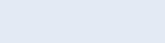# Standard Deviation Calculator

Home » Engineering » Standard Deviation Calculator

Standard deviation is a important tool in statistical and probabilistic analysis. Alone it is not sufficient to finding results and used along with other statistical tools.

It represents the spreadness of data, or dispersion of values in data relative to mean of the data. It is a measure of how each number is observed from the mean.

Square of Standard deviation is called Variance. It helps measure how far the data has spread out.

Concept of standard deviation applies only when data follows normal distribution. We can say data graph is in bell shape.

formula of standard deviationIn Statistics the statistical operations on whole data and a portion of it handled differently. Here comes the concept of sample and population. Complete or whole data is called as population i.e. the data which we are trying to analyse and make conclusions out of it. Part of the whole data is the sample.

How to calculate standard deviation:

• Calculate mean of the data. For this add all values in data and divide by size of data.
• Subtract mean from every value and square the each resultant value, then sum all.
• Divide the final value with size of data (N). If partial data is taken for calculation, then divide final value with size of data taken minus one (n-1) instead of N.
• Then apply square root, it is the standard deviation of the data.

By knowing the standard deviation one can get idea of the variation that is normal or drastic. Standard deviation used in the process of identifying the outliers of data i.e. unusual instances of data.

## Standard Deviation Example

### Students Marks in a Class

Calculating standard deviation of marks obtained by students in a class, who attend a exam. All marks are listed below in ascending order. No. of students in the class is 12. Maximum marks are 100, Pass marks are 50.

31, 42, 54, 56, 57, 63, 68, 75, 76, 82, 87, 91

Mean: 65.33, Std: 17.33

Lower and Upper standard deviation limits: 48, 82.33

0 —– 48 —– 65 —– 82.33 —– 100

Standard deviation of 17 marks tells that students performance varies significantly. Lower standard deviation spanned below into pass mark limit.